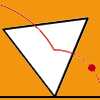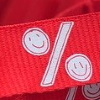# Resources tagged with: Interactivities

Filter by: Content type:
Age range:
Challenge level:

### There are 94 results

Broad Topics > Physical and Digital Manipulatives > Interactivities### Nine Colours

##### Age 11 to 16 Challenge Level:

Can you use small coloured cubes to make a 3 by 3 by 3 cube so that each face of the bigger cube contains one of each colour?### Estimating Angles

##### Age 7 to 14 Challenge Level:

How good are you at estimating angles?### Semi-regular Tessellations

##### Age 11 to 16 Challenge Level:

Semi-regular tessellations combine two or more different regular polygons to fill the plane. Can you find all the semi-regular tessellations?### Right Angles

##### Age 11 to 14 Challenge Level:

Can you make a right-angled triangle on this peg-board by joining up three points round the edge?### Sliding Puzzle

##### Age 11 to 16 Challenge Level:

The aim of the game is to slide the green square from the top right hand corner to the bottom left hand corner in the least number of moves.### Inside Out

##### Age 14 to 16 Challenge Level:

There are 27 small cubes in a 3 x 3 x 3 cube, 54 faces being visible at any one time. Is it possible to reorganise these cubes so that by dipping the large cube into a pot of paint three times you. . . .### The Triangle Game

##### Age 11 to 16 Challenge Level:

Can you discover whether this is a fair game?### Tilting Triangles

##### Age 14 to 16 Challenge Level:

A right-angled isosceles triangle is rotated about the centre point of a square. What can you say about the area of the part of the square covered by the triangle as it rotates?### Colour in the Square

##### Age 7 to 16 Challenge Level:

Can you put the 25 coloured tiles into the 5 x 5 square so that no column, no row and no diagonal line have tiles of the same colour in them?### Jam

##### Age 14 to 16 Challenge Level:

To avoid losing think of another very well known game where the patterns of play are similar.### Subtended Angles

##### Age 11 to 14 Challenge Level:

What is the relationship between the angle at the centre and the angles at the circumference, for angles which stand on the same arc? Can you prove it?### Icosian Game

##### Age 11 to 14 Challenge Level:

This problem is about investigating whether it is possible to start at one vertex of a platonic solid and visit every other vertex once only returning to the vertex you started at.### Square It

##### Age 11 to 16 Challenge Level:

Players take it in turns to choose a dot on the grid. The winner is the first to have four dots that can be joined to form a square.### Changing Places

##### Age 14 to 16 Challenge Level:

Place a red counter in the top left corner of a 4x4 array, which is covered by 14 other smaller counters, leaving a gap in the bottom right hand corner (HOME). What is the smallest number of moves. . . .### Triangles in Circles

##### Age 11 to 14 Challenge Level:

Can you find triangles on a 9-point circle? Can you work out their angles?### Just Rolling Round

##### Age 14 to 16 Challenge Level:

P is a point on the circumference of a circle radius r which rolls, without slipping, inside a circle of radius 2r. What is the locus of P?### Konigsberg Plus

##### Age 11 to 14 Challenge Level:

Euler discussed whether or not it was possible to stroll around Koenigsberg crossing each of its seven bridges exactly once. Experiment with different numbers of islands and bridges.### 3D Treasure Hunt

##### Age 14 to 18 Challenge Level:

Some treasure has been hidden in a three-dimensional grid! Can you work out a strategy to find it as efficiently as possible?### Olympic Magic

##### Age 14 to 16 Challenge Level:

in how many ways can you place the numbers 1, 2, 3 … 9 in the nine regions of the Olympic Emblem (5 overlapping circles) so that the amount in each ring is the same?### Magic W

##### Age 14 to 16 Challenge Level:

Find all the ways of placing the numbers 1 to 9 on a W shape, with 3 numbers on each leg, so that each set of 3 numbers has the same total.### Cogs

##### Age 11 to 14 Challenge Level:

A and B are two interlocking cogwheels having p teeth and q teeth respectively. One tooth on B is painted red. Find the values of p and q for which the red tooth on B contacts every gap on the. . . .### Excel Interactive Resource: Interactive Division

##### Age 11 to 16 Challenge Level:

Use an Excel to investigate division. Explore the relationships between the process elements using an interactive spreadsheet.### Interactive Number Patterns

##### Age 14 to 16 Challenge Level:

How good are you at finding the formula for a number pattern ?### More Number Pyramids

##### Age 11 to 14 Challenge Level:

When number pyramids have a sequence on the bottom layer, some interesting patterns emerge...### Rollin' Rollin' Rollin'

##### Age 11 to 14 Challenge Level:

Two circles of equal radius touch at P. One circle is fixed whilst the other moves, rolling without slipping, all the way round. How many times does the moving coin revolve before returning to P?### A Tilted Square

##### Age 14 to 16 Challenge Level:

The opposite vertices of a square have coordinates (a,b) and (c,d). What are the coordinates of the other vertices?### Disappearing Square

##### Age 11 to 14 Challenge Level:

Do you know how to find the area of a triangle? You can count the squares. What happens if we turn the triangle on end? Press the button and see. Try counting the number of units in the triangle now. . . .### Number Pyramids

##### Age 11 to 14 Challenge Level:

Try entering different sets of numbers in the number pyramids. How does the total at the top change?### Got It

##### Age 7 to 14 Challenge Level:

A game for two people, or play online. Given a target number, say 23, and a range of numbers to choose from, say 1-4, players take it in turns to add to the running total to hit their target.### Picturing Triangular Numbers

##### Age 11 to 14 Challenge Level:

Triangular numbers can be represented by a triangular array of squares. What do you notice about the sum of identical triangle numbers?### Partitioning Revisited

##### Age 11 to 14 Challenge Level:

We can show that (x + 1)² = x² + 2x + 1 by considering the area of an (x + 1) by (x + 1) square. Show in a similar way that (x + 2)² = x² + 4x + 4### Bow Tie

##### Age 11 to 14 Challenge Level:

Show how this pentagonal tile can be used to tile the plane and describe the transformations which map this pentagon to its images in the tiling.### Excel Interactive Resource: Fraction Multiplication

##### Age 11 to 16 Challenge Level:

Use Excel to explore multiplication of fractions.### Factors and Multiples Game

##### Age 7 to 16 Challenge Level:

A game in which players take it in turns to choose a number. Can you block your opponent?### Isosceles Triangles

##### Age 11 to 14 Challenge Level:

Draw some isosceles triangles with an area of $9$cm$^2$ and a vertex at (20,20). If all the vertices must have whole number coordinates, how many is it possible to draw?### Got it Article

##### Age 7 to 14

This article gives you a few ideas for understanding the Got It! game and how you might find a winning strategy.### Excel Interactive Resource: the up and Down Game

##### Age 11 to 16 Challenge Level:

Use an interactive Excel spreadsheet to explore number in this exciting game!### Excel Interactive Resource: Number Grid Functions

##### Age 11 to 16 Challenge Level:

Use Excel to investigate the effect of translations around a number grid.### Square Coordinates

##### Age 11 to 14 Challenge Level:

A tilted square is a square with no horizontal sides. Can you devise a general instruction for the construction of a square when you are given just one of its sides?### Excel Interactive Resource: Multiples Chain

##### Age 11 to 16 Challenge Level:

Use an interactive Excel spreadsheet to investigate factors and multiples.### Excel Interactive Resource: Fraction Addition & Fraction Subtraction

##### Age 11 to 16 Challenge Level:

Use Excel to practise adding and subtracting fractions.### Excel Interactive Resource: Haitch

##### Age 11 to 16 Challenge Level:

An Excel spreadsheet with an investigation.### Excel Interactive Resource: Equivalent Fraction Bars

##### Age 11 to 16 Challenge Level:

A simple file for the Interactive whiteboard or PC screen, demonstrating equivalent fractions.### Excel Interactive Resource: Long Multiplication

##### Age 11 to 16 Challenge Level:

Use an Excel spreadsheet to explore long multiplication.### Tessellation Interactivity

##### Age 7 to 16 Challenge Level:

An environment that enables you to investigate tessellations of regular polygons### Speeding Up, Slowing Down

##### Age 11 to 14 Challenge Level:

Experiment with the interactivity of "rolling" regular polygons, and explore how the different positions of the red dot affects its speed at each stage.### Fractions and Percentages Card Game

##### Age 11 to 16 Challenge Level:

Can you find the pairs that represent the same amount of money?### Got a Strategy for Last Biscuit?

##### Age 11 to 16 Challenge Level:

Can you beat the computer in the challenging strategy game?### Stars

##### Age 11 to 14 Challenge Level:

Can you find a relationship between the number of dots on the circle and the number of steps that will ensure that all points are hit?### First Connect Three

##### Age 7 to 14 Challenge Level:

Add or subtract the two numbers on the spinners and try to complete a row of three. Are there some numbers that are good to aim for?